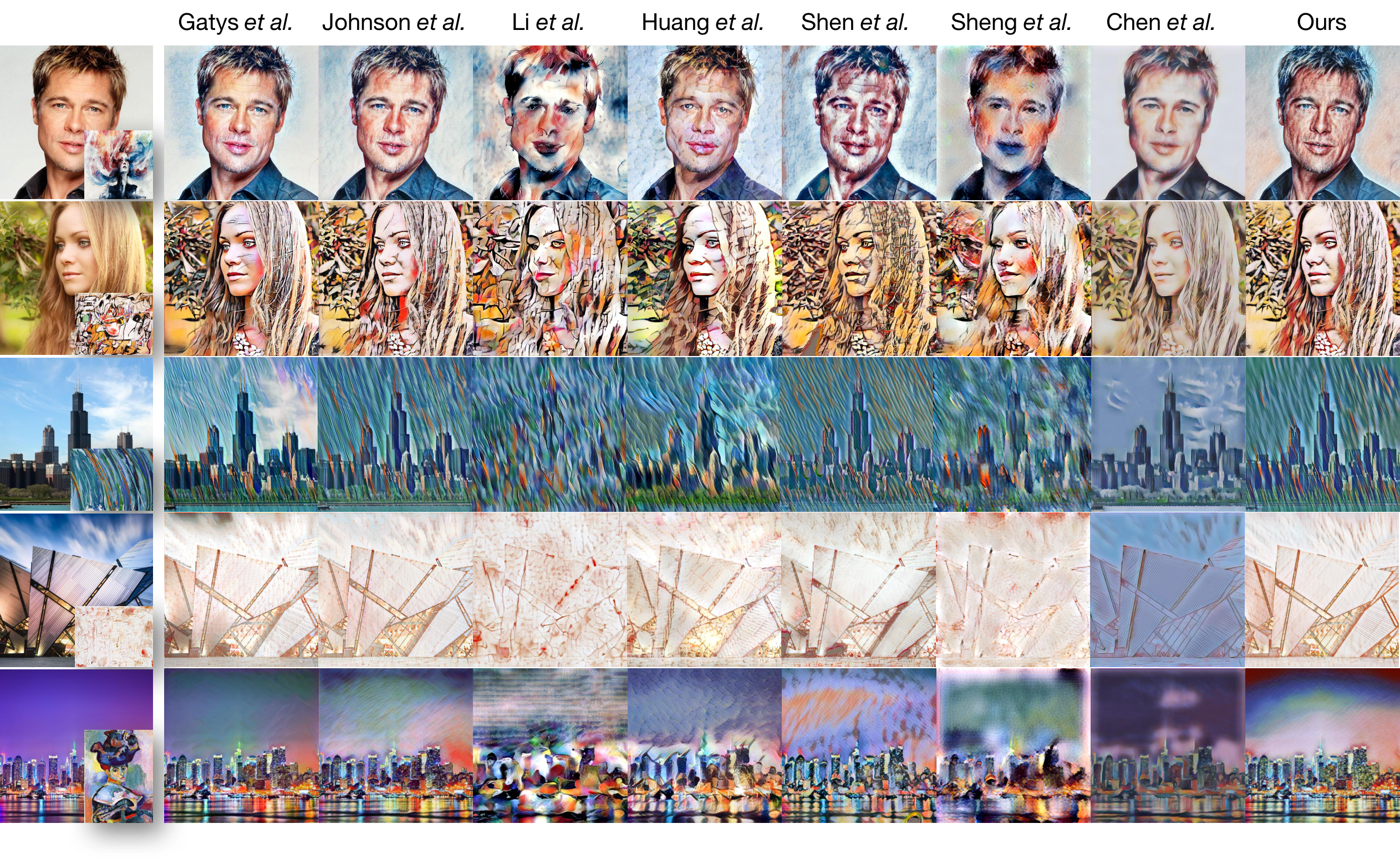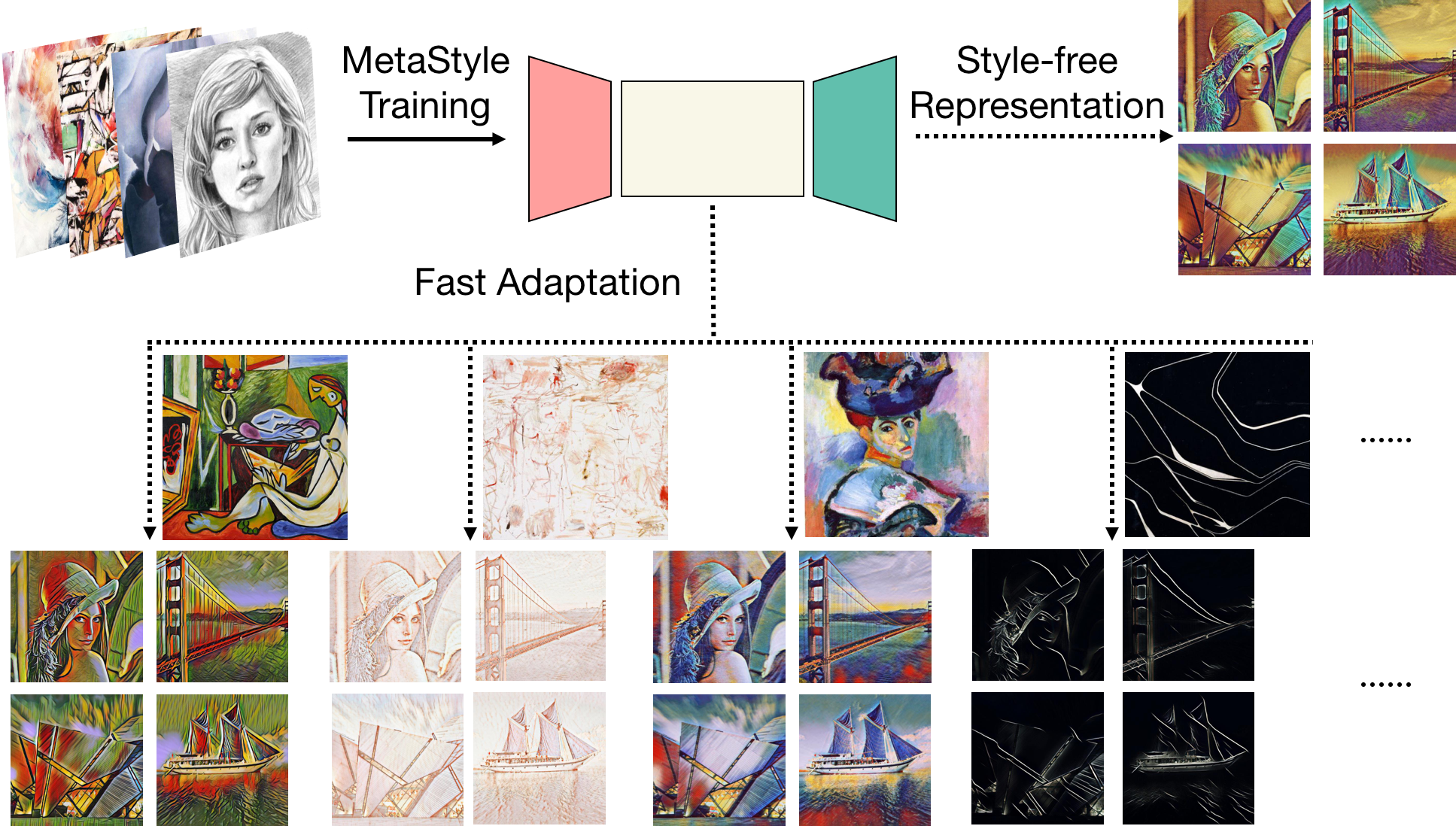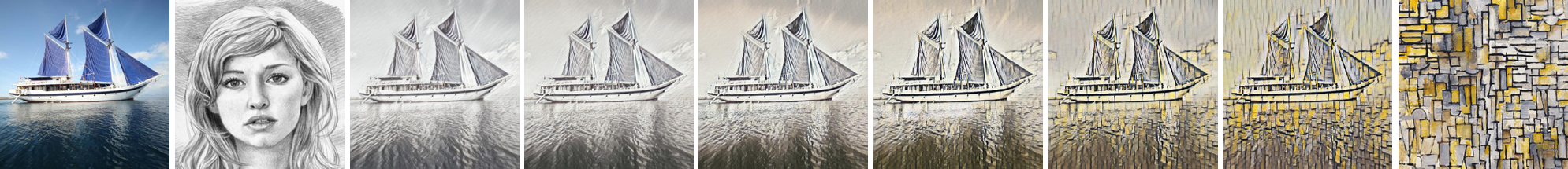# MetaStyle: Trading Off Speed, Flexibility, and Quality in Neural Style Transfer

Posted by Chi Zhang on December 6, 2018

This post briefly summarizes our work on trading off speed, flexibility, and quality in neural style transfer. For further details, please refer to our AAAI 2019 paper.Figure 1. Stylization comparison between our method and others.

Figure 2. Frame-by-frame video stylization result of MetaStyle.

## 1. Introduction

We have witnessed an unprecedented booming in the research area of artistic style transfer ever since Gatys et al.  introduced the neural method. However, there is still one remaining challenge in this area: how to balance a trade-off among three critical aspects of neural style transfer algorithms—speed, flexibility, and quality:

• The vanilla optimization-based algorithm produces impressive results for arbitrary styles, but is unsatisfyingly slow due to its iterative nature.
• The fast approximation methods based on feed-forward neural networks generate satisfactory artistic effects but bound to only a limited number of styles.
• Feature-matching methods like AdaIN achieve arbitrary style transfer in a real-time manner but at a cost of the compromised quality.

We find it considerably difficult to balance the trade-off well merely using a single feed-forward step and try to find, instead, an algorithm that could adapt quickly to any style, while the adapted model maintains high efficiency and good image quality.

Motivated by this idea, we propose a novel method, coined MetaStyle, which formulates the neural style transfer as a bilevel optimization problem and combines learning with only a few post-processing update steps to adapt to a fast approximation model with satisfying artistic effects, comparable to the optimization-based methods for an arbitrary style. The qualitative and quantitative analysis in the experiments demonstrate that the proposed approach achieves high-quality arbitrary artistic style transfer effectively, with a good trade-off among speed, flexibility, and quality.

In summary:

• The unique problem formulation encourages the model to learn a style-free representation for content images, and to produce a new feed-forward model, after only a small number of update steps, to generate high-quality style transfer images for a single style efficiently. From another perspective, this formulation could also be thought of as finding a style-neutral input for the vanilla optimization-based methods, but transferring styles much more effectively.
• Our model is instantiated using a neural network. The network structure is inspired by the finding that scaling and shifting parameters in instance normalization layers are specialized for specific styles. In contrast, unlike prior work, our method implicitly forces the parameters to find no-style features in order to rapidly adapt and remain parsimonious in terms of the model size. The trained MetaStyle model has roughly the same number of parameters as described in Johnson et al. , and requires merely 0.1 million training steps.

## 2. Background

#### 2.1. Style Transfer and Perceptual Loss

Given an image pair $$(I_c, I_s)$$, the style transfer task aims to find an “optimal” solution $$I_x$$ that preserves the content of $$I_c$$ in the style of $$I_s$$. Gatys et al. proposed to measure the optimality with a newly defined loss using the trained VGG features, later modified and named as the perceptual loss. The perceptual loss could be decomposed into two parts: the content loss and the style loss.

Denoting the VGG features at layer $$i$$ as $$\phi_i(\cdot)$$, the content loss $$\ell_{\text{content}}(I_c, I_x)$$ is defined using the $$L_2$$ norm

$\ell_{\text{content}}(I_c, I_x) = \frac{1}{N_i} ||\phi_i(I_c) - \phi_i(I_x)||_2^2,$

where $$N_i$$ denotes the number of features at layer $$i$$.

The style loss $$\ell_{\text{style}}(I_s, I_x)$$ is the sum of Frobenius norms between the Gram matrices of the VGG features at different layers

$\ell_{\text{style}}(I_s, I_x) = \sum_{i \in S} ||G(\phi_i(I_s)) - G(\phi_i(I_x))||_F^2,$

where $$S$$ denotes a predefined set of layers and $$G$$ the Gramian transformation.

The transformation could be efficiently computed by

$G(x) = \frac{\psi(x) \psi(x)^T}{C H W}$

for a 3D tensor $x$ of shape $$C \times H \times W$$, where $$\psi(\cdot)$$ reshapes $$x$$ into $$C \times H W$$.

The perceptual loss $$\ell(I_c, I_s, I_x)$$ aggregates the two components by the weighted sum

$\ell(I_c, I_s, I_x) = \alpha \ell_{\text{content}}(I_c, I_x) + \beta \ell_{\text{style}}(I_s, I_x).$

#### 2.2. Bilevel Optimization

We formulate the style transfer problem as the bilevel optimization in the simplified form

\begin{equation} \begin{aligned} & \underset{\theta}{\text{minimize}} & & E(w_\theta, \theta) \\ & \text{subject to} & & w_\theta = \text{argmin}_w L_\theta(w), \end{aligned} \end{equation}

where $$E$$ is the outer objective and $$L_\theta$$ the inner objective. Under differentiable $$L_\theta$$, the constraint could be replaced with $$\nabla L_\theta = 0$$. However, in general, no closed-form solution of $$w_\theta$$ exists and a practical approach to approximate the optimal solution is to replace the inner problem with the gradient dynamics, i.e.,

\begin{equation} \begin{aligned} & \underset{\theta}{\text{minimize}} & & E(w_T, \theta) \\ & \text{subject to} & & w_0 = \Psi(\theta) \\ & & & w_{t} = w_{t - 1} - \delta \nabla L_\theta(w_{t - 1}) \end{aligned} \end{equation}

where $$\Psi$$ initializes $$w_0$$, $$\delta$$ is the step size and $$T$$ the maximum number of steps.

## 3. MetaStyle

As discussed in the previous section, we choose $$\theta$$ to be our model initialization and $$w_T$$ the adapted parameters, denoted as $w_{s, T}$ to emphasize the style to adapt to. $$T$$ is restricted to be small, usually in the range between 1 and 5. Both the inner and outer objective is designed to be the perceptual loss averaged across datasets. We further use an identity mapping for $$\Psi$$. Now, the problem could be stated as

\begin{equation} \begin{aligned} &\underset{\theta}{\text{minimize}} & & \mathbb{E}_{c,s}[\ell(I_c, I_s, M(I_c; w_{s, T}))] \\ &\text{subject to} & & w_{s, 0} = \theta \\ & & & w_{s, t} = w_{s, t - 1} - \delta \nabla \mathbb{E}_c[\ell(I_c, I_s, M(I_c; w_{s, t - 1}))], \end{aligned} \end{equation}

where $$M(\cdot; \cdot)$$ denotes our model and $$\delta$$ the learning rate of the inner objective. The expectation of the outer objective $$\mathbb{E}_{c,s}$$ is taken with respect to both the style and the content images in the validation set, whereas the expectation of the inner objective $$\mathbb{E}_c$$ is taken with respect to the content images in the training set only. This design allows the adapted model to specialize for a single style but still maintain the initialization generalized enough. Note that for the outer objective, $$w_{s, T}$$ implicitly depends on $$\theta$$. In essence, the framework learns an initialization $$M(\cdot; \theta)$$ that could adapt to $$M(\cdot; w_{s, T})$$ efficiently and preserve high image quality for an arbitrary style. Figure 2 shows the proposed framework.Figure 3. The proposed MetaStyle framework, in which the model is optimized using the bilevel optimization over large-scale content and style dataset. The framework first learns a style-neutral representation. A limited number of post-processing update steps is then applied to adapt the model quickly to a new style. After adaptation, the new model serves as an image transformation network with good transfer quality and high efficiency.

For adaption, we post update our model initialized with $$\theta$$, i.e., $$M(\cdot; \theta)$$ for only a limited number of steps (usually 100 to 200 steps suffice) and the model becomes tailored to a specific style.

## 4. Experiment

We train our model using MS-COCO as our content image dataset and WikiArt as our style image dataset. We follow common practice in conducting experiments and would like to show our results in the following.

#### 4.1. Speed and Flexibility

Method Param 256 (s) 512 (s) # Styles
Gatys et al. N/A 7.7428 27.0517 $$\infty$$
Johnson et al. 1.68M 0.0044 0.0146 1
Li et al. 34.23M 0.6887 1.2335 $$\infty$$
Huang et al. 7.01M 0.0165 0.0320 $$\infty$$
Shen et al. 219.32M 0.0045 0.0147 $$\infty$$
Sheng et al. 147.22M 0.5089 0.6088 $$\infty$$
Chen et al. 1.48M 0.2679 1.0890 $$\infty$$
Ours 1.68M 0.0047 0.0145 $$\infty^\star$$

Table 1. Speed and flexibility benchmarking results. Param lists the number of parameters in each model. 256/512 denotes inputs of 256 $$\times$$ 256/512 $$\times$$ 512. # Styles represents the number of styles a model could potentially handle. $$^\star$$Note that MetaStyle adapts to a specific style after very few update steps and the speed is measured for models adapted.

Table 1 summarizes the benchmarking results regarding style transfer speed and model flexibility. As shown in the table, our method achieves the same efficiency as Johnson et al. and Shen et al.. Additionally, unlike Shen et al. that introduces a gigantic parameter prediction model, MetaStyle is parsimonious with roughly the same number of parameters as Johnson et al.. While Johnson et al. requires training a new style model from scratch, MetaStyle could be immediately adapted to any style with a negligible number of updates under 30 seconds. This property significantly reduces the efforts in arbitrary style transfer and, at the same time, maintains a high image generation quality, as shown next.

#### 4.2. Quality

Figure 1 shows the qualitative comparisons of the style transfer between the existing methods and the proposed MetaStyle method. We notice that, overall, Gatys et al. and Johnson et al. obtain the best image quality among all the methods we tested. This observation coheres with our expectation, as Gatys et al. iteratively refines a single input image using an optimization method, whereas the model from Johnson et al. learns to approximate optimal solutions after seeing a large number of images and a fixed style, resulting in a better generalization.

Among methods capable of arbitrary style transfer, Li et al. applies style strokes excessively to the contents, making the style transfer results become deformed blobs of color, losing much of the image structures in the content images. Looking deep into Huang et al., we notice that the arbitrary style transfer method produces images with unnatural cracks and discontinuities. Results from Shen et al. come with strange and peculiar color regions that likely result from non-converged image transformation models. Sheng et al. unnecessarily morphs the contours of the content images, making the generated artistic effects inferior. The inverse network from Chen et al. seems to apply the color distribution in the style image to the content image without successfully transferring the strokes and artistic effects in style.Figure 4. Two-style interpolation results. The content image and style images are shown on the two ends.

• Style Interpolation: To interpolate among a set of styles, we perform a convex combination on the parameters of adapted MetaStyle models. Figure 3 shows the results of a two-style interpolation.
• Video Style Transfer: We perform the video style transfer by first training the MetaStyle model for a small number of iterations to adapt to a specific style, and then applying the transformation to a video sequence frame by frame. Figure 2 shows the video style transfer results in five consecutive frames. Note that our method does not introduce the flickering effect that harms aesthetics.

## 5. Conclusion

We present the MetaStyle, a model designed to achieve a right three-way trade-off among speed, flexibility, and quality in neural style transfer. Unlike previous methods, MetaStyle considers the arbitrary style transfer problem in a new scenario where a small (even negligible) number of post-processing updates are allowed to adapt the model quickly to a specific style. In experiments, we show that MetaStyle could adapt quickly to an arbitrary style within a small number iterations. Each adapted model is an image transformation network and benefits the high efficiency and style transformation quality on par with Johnson et al.. These results show MetaStyle indeed achieves a right trade-off.

@inproceedings{zhang2019metastyle,